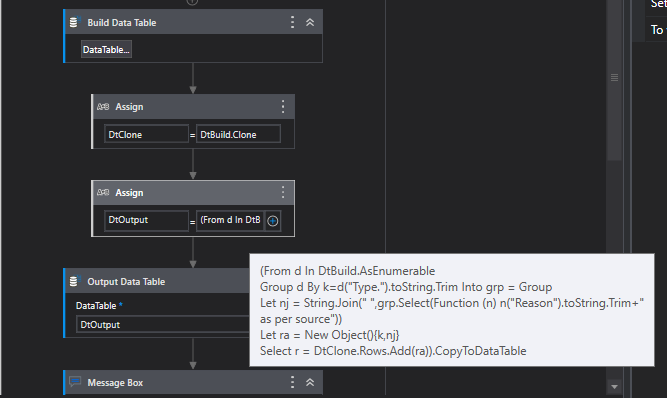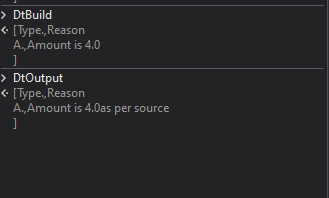# How to append the value in datatable of particular row

For example

Type. Reason

A. Amount is 4.0

I need to add " as per source" in the reason, my output will be

Type. Reason

A. Amount is 4.0 as per source

To append the value in a DataTable in UiPath, you can use the “AddDataRow” method of the DataTable object. This method allows you to add a new row to the data table with the specified values.

Can you explain in detail

Try with this expression

``````(From d In DTInput1.AsEnumerable
Group d By k=d("Type.").toString.Trim Into grp = Group
Let nj = String.Join(" ",grp.Select(Function (n) n("Reason").toString.Trim+"as per source"))
Let ra = New Object(){k,nj}
``````

Regards
Gokul

Check out the XAML file

LinqGroupby.xaml (13.0 KB)OutputRegards
Gokul

As same like this expression i shared in your other post you just need to modify the value inside the quotes

Use this expression

``````(From row In Dt_inp.AsEnumerable
Group row By sc = row("Type").ToString()
Into grp = Group
Let total= grp.sum(Function (x) Convert.ToDouble(System.Text.RegularExpressions.Regex.Match(x("Reason").ToString,"\d+\.\d+").ToString))
Let type=grp(0)("Type").ToString
Let result = New Object() {type, "Amount is "+CDec(total).ToString("F1")+" as per source"}